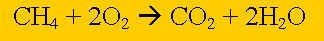Number-Number stoichiometryMethane burns in oxygen to produce carbon dioxide and water according to the equation above. If 1.20 X 1023 number of methane molecules completely burn in excess oxygen calculate the number of water molecules formed. Step 1 Calculate the number of mole of methane present. Step 2 Calculate the ratio from the prefixes in the balanced equation above Step 3 Calculate the number of mole of water produced. Step 4 Calculate the number of water molecules produced.

Home